10. MODELS OF THE ATOMS AND THE MOLECULES

# The mathematical model of the law of formation of the spectra of the atoms and the ions (207) proves the lack of orbital motion of the electrons in the atoms. The atomic nucleus models built by us confirm this fact.

The protons in the atomic nuclei are arranged on their surface and have a free magnetic pole for the connection with the like magnetic pole of the electron. The chain of consistency of actions of Nature determined in this search confirms adjacency of the models of the electron, the proton and neutron to reality. That’s why we can get ahead and begin to analyze the structure of the atoms of chemical elements. The hydrogen atom is the first and the simplest atom. Let us check the ideas described by us taking its formation as an example.

10.2. Structure of the Hydrogen Atom

The largest array of experimental information concerning the hydrogen atom is in its spectra , . In order to determine the structure of the hydrogen atom, we should analyze this information. We have already given the calculation of the spectrum of the hydrogen atom. As the hydrogen atom model is built on the basis of this calculation, let us give this calculation one again. First of all. Let us calculate the energies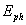of the photons radiated and absorbed by the electron during its transitions and the binding energies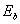of the electron with the atomic nucleus (proton), which correspond to these transitions.

In order to calculate the energies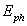of the photons absorbed or radiated by the electron during its transitions, we’ll use the spectrum formation law discovered by us  (207) , ,  and the experimental spectroscopy data , .

We’ll calculate binding energyof the electron with the nucleus, which corresponds to any energy level, according to the formula (206).

Taking into consideration the fact that ionization energy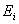of the hydrogen atom is equal to binding energy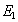of the electron with the nucleus, which corresponds to the first energy level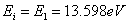and using the formulas (212) and (213), we’ll get the energies of the photons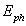(theor.) radiated or absorbed by the electron and binding energies(theor.) of the electron with the atomic nucleus, which correspond to n-energy levels (Table 27).

Table 27. Spectrum of the hydrogen atom

 Values n 2 3 4 5 6(exper.) eV 10.2 12.09 12.75 13.05 13.22(theor.) eV 10.198 12.087 12.748 13.054 13.22(theor.) eV 3.4 1.51 0.85 0.54 0.38

It follows from the spectroscopy law (212) that the energies of absorbed and radiated photons during the transition of the electron between the energy levels n and n+1 are calculated according to the formula (216).

Let us list the results of the calculation (Table 6) of the energies of the photons(theor.) radiated or absorbed by the electron of the hydrogen atom during transitions between the levels n and n+1 as compared with the experimental(exper.) data.

Table 28. Energies of interlevel transitions of the electron of the hydrogen atom

 Levels n, n+1 2...3 3...4 4...5 5...6 6...7 7...8(exper) eV 1.89 0.66 0.30 0.17 0.10 0.07(theor.) eV 1.88 0.661 0.306 0.166 0.100 0.065

We should note that binding energiesof the electron with the atomic nucleus are specified in Table 27. Other energies of the radiated or absorbed photons during the transitions of the electron are given in Table 28.

The formula (216) gives the possibility to calculate the energies of the radiated and absorbed photons during any transitions of the electron. For example, when the electron goes from the 1st to the 10th energy level, it absorbs the photon with the energy, which is calculated according to the formula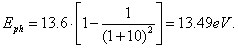(236)

If the electron goes from, say, the 15th to the 10th energy level, it radiates the photon with energy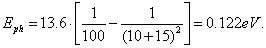(237)

Let us see how free electron energy will be changed during absorption and emission of the photons. As ionization energy of the hydrogen atom is equal to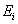=13.598 eV, it means that when the free electron is united with the nucleus of the hydrogen atom (the proton), it should emit the photon with the energy being equal to ionization energy  and occupy the first (n=1) non-exited energy level. Its full energy 511.100 eV is reduced and becomes equal to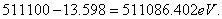(238)

Then, after absorption of the photon with energy of 10.20 eV and the transfer to the second energy level the complete energy of the electron will be increased and will become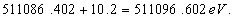(239)

Thus, during the transitions to higher energy levels the electron increasing its full energy due to the absorbed photons approximates its value to the energy value, which it has in free state. This increase takes place at the expense of the absorbed photons, and binding energyof the electron with the proton being decreased approximates to zero (Table 27), and it becomes free. All electrons of any atom act in such a way, and its allows them to be in free state with one and the same mass and with one and the same charge. That’s why mass and charge of all free electrons are always constant.

Thus, we have agreed that free electron energy is equal to its photon energy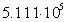. It means that in order to calculate radius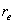of the electron when it is on the first energy level in the hydrogen atom, it is necessary to take free electron energy and to subtract the energy, which it has radiated in the form of the photon, from it. During the determination of the electron radius when it is at the first energy level, this energy is equal to 522100-13.6=511086.4 eV. The value of the electron radius will be as follows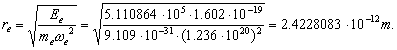(240)

This value differs from the value, which is calculated taking into consideration full energy of the electron (138) for nominal value of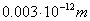.

It is clear that the energy and, consequently, the electron radius in the atom are changed insufficiently, and this fact matches the constant value of Compton wavelength of the electron, which now we should call Compton radius of the electron.

We have already shown that photon mass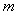, radius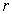and frequency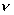are changed in such a way that their product (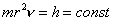) remains constant, because the change of a set of the above-mentioned parameters is governed by the law of conservation of angular momentum , . The same law governs a change of the same set of the parameters of the electron.

Let us pay attention to one more important fact. Binding energy of the electron with the nucleus is changed in proportion to the change of square of frequency. But if we consider the dependence of frequency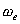of electron rotation relative to its axis of symmetry from strength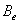of magnetic field of the electron (148), one more reason of the change of electron bond energy with the nucleus takes place during its energy transition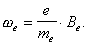(241)

As angular rotational speed of the electron is proportional to its magnetic field strength, we substitute strengthof its magnetic field for electron rotational speedin the mathematical expression, which determines electron energy,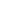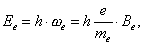(242)

and get the same result, but with another physical meaning: electron energy is aliquot not to angular frequency, but to strengthof magnetic field of the electron. It is an important consequence. It points out to possible lack of rotation of the electron in relation to its axis in the  moment when it is in the atom.

We’d like to draw our readers’ attention to the result of the joint solution of the relations (14), (18), (148), (150) and (241)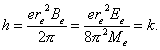(243)

Is it a new constant?

Hydrogen is the simplest atom. It has one electron, and its nucleus consists of one proton. The information found out by us concerning the structure of the electron and proton gives the opportunity to get a notion about the formation process of this atom .

One can suppose that magnetic fields of both the proton and the electron are similar to magnetic fields of the bar magnets and have magnetic poles. As proton mass is much more greater than electron mass, the hydrogen mass formation will begin with the convergence of the electron to the proton. We know that in free sate the electron has magnetic moment and rather large magnetic field strength in its geometrical centre, that’s why both electrical force and magnetic forces will govern the process of the convergence of the electron with the proton at the first stage.

As the magnetic fields of both the proton and the electron have the largest strength about their axes of rotation, the electron and the proton will rotate align during the convergence. If their opposite magnetic poles are directed to meet each other, both the electrical forces and the magnetic ones will draw the electron and the proton together, and the proton will absorb the electron and will become a neutron. As the results obtained by the Japanese investigators have shown , this process is realized during plasma electrolysis of water. When the electron is drawn together with the proton and their like magnetic poles are directed to meet each other, Coulomb’s forces acting not along the convergence axis, but normally to toroidal surface of the electron will draw it together with the proton, and magnetic forces will repulse them from each other. An equilibrium will be set between these forces, and the structure being formed is such a way is the hydrogen atom (Fig. 50). This phenomenon is confirmed by the results of the investigations of plasma electrolysis of water .

A question arises: how does the electron move in relation to the atomic nucleus if it has no orbital movement? As the law (88, 214, 215) reflects the electron rotation only in relation to its axis, the electron with the nucleus can interact only by the axis of rotation. It can be presented in the form of a rotating peg-top, which electromagnetic axis of rotation rests on the atomic nucleus, and it rotates in relation to its axis of symmetry (Fig. 50).

The nature has made the electron in such a way that it has electric field, which form is similar to a spherical one and magnetic field is similar to magnetic field of the bar magnet. The forces of unlike electrical fields bring the electron close to the atomic nucleus, and the forces of their like magnetic poles limit this approximation.

Thus, it appears from the results of our investigations that the atomic nucleus has the size by a factor of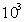less than the size of the electron. It is arranged on its axis, at a certain distance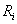from the geometrical centre of the electron, which can be calculated taking Coulomb’s law as the base. It gives the opportunity to determine the distance between the proton and the electron when it is on the first energy level. As the binding energy of the proton with the electron is equal to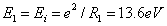in this case, we’ll have the following expression when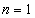.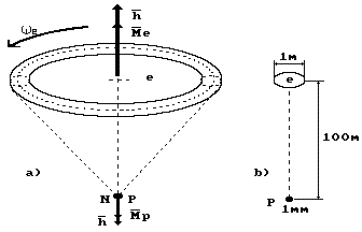Fig. 50. Diagram of the hydrogen atom model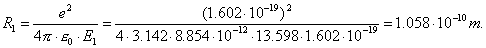(244)

The calculation resultsfor n=2,3,4…. are given in Table 29.

Table 29. Spectrum of the hydrogen atom, its binding energybetween the proton and the electron and distancesbetween them

 Volumes n 2 3 4 5 6(exper) eV 10.2 12.09 12.75 13.05 13.22(theor) eV 10.198 12.087 12.748 13.05 13.22(theor) eV 3.4 1.51 0.85 0.54 0.38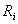(теор)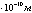4.23 9.54 16.94 26.67 37.89

Minimal distance, at which the electron comes to the proton, is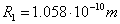(234), i.e. of the order of Angstrom; it agrees with the hydrogen atom size accepted in modern physics (Fig. 50).

If we accept that the size of the proton is equal to one millimeter, the size of the electron will be nearly one meter, and the distance between the nucleus of the hydrogen atom (the proton) and the electron will be equal to one hundred meters (Fig. 50, b).

In Fig. 51, a diagram of the consecutive transition of the electron of the hydrogen atom from the first energy level (I) to the second one (II), from the second one to the third one (III) and from the third one to the fourth one (IV) is shown.

As it is clear, binding energy of the electron with the proton P at the moment of its stay at the first (I) energy level is equal to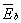=13.6 eV, and full energy is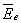=5.11×105 eV. After absorption of the photon with energy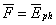=10.2 eV, the electron goes to the second (II) energy level, and its binding energy becomes equal to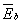=3.4 eV. When the photon with energy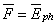=1.89 eV is absorbed, the electron goes to the third (III) energy level, and its binding energy with the nucleus becomes equal to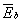=1.51 eV, etc.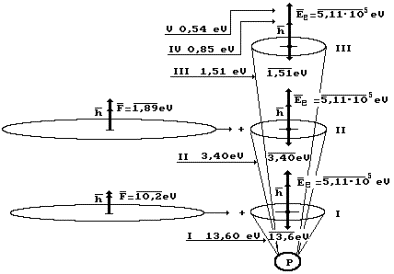Fig. 51. Diagram of addition of vectors of energies of the photon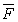, the electron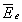and binding energy of the electron with the atomic nucleus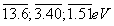… in the absorption processes; I, II, III… are energy levels of the electron

When binding energywit the electron with the nucleus is increased, it comes nearer to the atomic nucleus or is submerged deeper into its cell. We understand ‘the cell” as a volume of a conical form with the apex at the atomic nucleus, in which the electron rotates like the peg-top. The larger the binding energy of the electron with the nucleus, the nearer it is situated to the nucleus or the deeper in its cell.

When the photons are absorbed, binding energy of the electron with the nucleus is reduced, and it goes on rotating and precessing on the nucleus and with draws  from it coming nearer to the surface of the atom. When the electron emits the photons, its binding energy with the atomic nucleus is increased, and it is submerged deeper in its “cells”.

It is important that there are no trigonometric functions in the mathematical expression (212) of the spectrum formation law. It means that the processes of absorption and emission of the photons by the electrons take place only in case when vectors of their single energies are directed along one straight line. If it were not the case, the correction factors or trigonometric functions would be present in the spectrum formation law of the atoms and ions. But we cannot exclude the possibility of emergence of these factors and trigonometric functions during the calculation of the spectra of the atoms with many electrons and the ions.

Thus, the hydrogen atom is a core with the positively charge proton at one end and the negatively charged electron at the second end. The size of the electron is by a factor of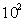less than the size of the atom itself, and the size of the proton is by a factor ofless than the size of the electron and by a factor of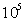less than the size of the atom (Fig. 50, b).

As magnetic moment of the electron (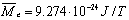) is by a factor of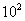greater than the magnetic moment of the proton (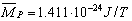) and as their vectors in the hydrogen atom are directed in the opposed ways, magnetic moment of the hydrogen atom is practically equal to magnetic moment of the electron or Bohr magneton`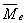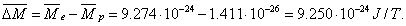(245)

Besides, equality of spins of the electron and the proton and their opposite directivity make the hydrogen atom spin equal to zero.

The hydrogen atom model shown in Fig. 50 demonstrates its activity. On the one hand, there is a positively charged proton, which is ready to be connected with a free electron; on the other hand, there is a negatively charged electron, which is ready to be connected with a proton or an electron. That’s why the hydrogen atoms can exist only at high temperature (5000…10000)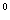C. At this temperature the atomic electrons are in exited state, i.e. at the highest energy levels where their bond with the protons is very weak.

The cause of the emission of the photons during approximation of the electron with the proton can be described in the following way. During the coaxial approximation of the electron with the proton different numbers of their revolution in relation to one and the same axis form the moment of forces, which will inhibit or accelerate rotation of the electron. In the initial stage, the moments of forces of the proton and the electron will be different. In order to restore equality of these moments, the electron emits a part of its mass in the form of the photon or absorbs it from the environment and is brought nearer or comes off from the proton.

Here one should take into account the photon condition of the environment. If the concentration of the photons, which are necessary for the electron for the transition to the next energy level, is enough, it will absorb such photon and make the corresponding energy transition. If there is a lack of the photons, which correspond to the existence of the electron at the definite energy level, in the environment, it will emit this photon and will move to lower energy level. The necessary equilibrium of the photons is preserved in the environment, which determines its temperature. It is necessary to analyze photon pressure on everything that exists in the photon environment in the same way as, for example, in liquid or gaseous medium, but we’ll not consider this necessity, because there exist more interesting tasks.

The Foundations of Physchemistry of Microworld

Ó2003 Kanarev Ph. M.

Internet Version - http://book.physchemistry.innoplaza.net

<< Back to Physchemistry Book Index